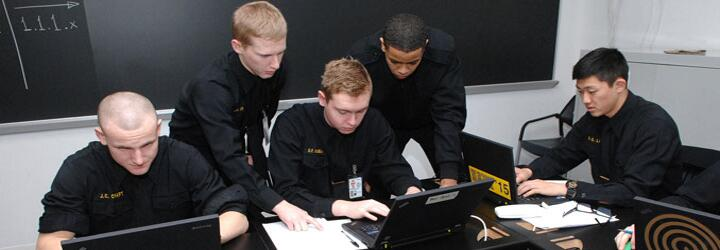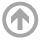Mathematics Department# Math Major

## Introduction

The mathematical challenges facing the twenty-first century Navy touch a vast number of sub-disciplines in mathematics, from the traditional applied math subjects of numerical analysis, engineering mathematics, operations research , and statistics to problems applying discrete math, algebra, and algebraic geometry. The Mathematics Major also provides an excellent foundation for graduate work in any technical field as well as in economics, business, or law. The USNA Mathematics Department now offers two tracks, in order to provide midshipmen more in-depth study in a particular area of mathematics, culminating in a capstone project in the first-class year. Both tracks teach midshipmen to think logically and critically and provide excellent background for further study in mathematics and the sciences. Honors programs are available in both tracks.

### Applied Mathematics Track

The Applied Mathematics Track focuses on modeling, estimation, and numerical approximation methods. The primary emphasis of this track is modeling of real-world problems. Students study methods used to obtain numerical results to various problems and analyze (or predict) the errors produced by those approximations. Students have the opportunity to learn about Navy applications. Mathematics has been a significant enabler in the DoD's Autonomous Vehicle Program, specifically the drone program, by developing techniques for image segmentation and automatic target recognition. Algorithm development for inverse problems especially in the areas of underwater acoustics and electromagnetics continues to play a critical role in enhancing DoN's capabilities in signal processing and sensor development. Mathematics is a key tool in DoD's laser research in the fields of laser communication and directed energy. Mathematics is a pivotal scientific component in our understanding of ocean currents, weather prediction and climate change, all established areas of need for the Navy. The mathematics department offers special topics courses, capstone and honors projects, and Bowman and Trident Scholar research on these and other applications.

### Mathematics Track

The Mathematics Track offers more background in theory and foundations of mathematics, with courses in the traditional areas of analysis, linear algebra, complex variables, algebra, topology, geometry, and combinatorics, applications such as cryptography and coding theory, and special topics courses such as differential geometry, algebraic geometry, and mathematical physics.

## Learning Outcomes

USNA graduates in Applied Mathematics are able to…

• Call on standard mathematical tools from a variety of applicable fields of mathematics. These tools include partial differential equations, probability, linear algebra, numerical analysis, and higher level computer programming. (“Preparation”)
• Mathematically model natural phenomena. (“Formulation”)
• Rigorously apply mathematical methods to effectively analyze mathematical models and draw conclusions from them. (“Analysis”)
• Understand the limits of mathematical models and of computational methods, and whether they’ve led to reasonable results. (“Evaluation”)
• Communicate effectively in plain English and with technical precision, in both written and oral presentations. (“Communication”)

USNA graduates in Mathematics are able to…

• Call on ideas, definitions, structures, and theorems from a variety of mathematical fields. These fields include algebra, real analysis, and linear algebra. (“Preparation”)
• Investigate mathematical definitions and statements, supplying useful examples, relevant theorems, and possible avenues of proof. (“Formulation”)
• Devise simple proofs and follow more complicated proofs of mathematical statements. (“Analysis”)
• Determine whether arguments are valid and conclusions are reasonable. (“Evaluation”)
• Communicate effectively in plain English and with technical precision, in both written and oral presentations. (“Communication”)